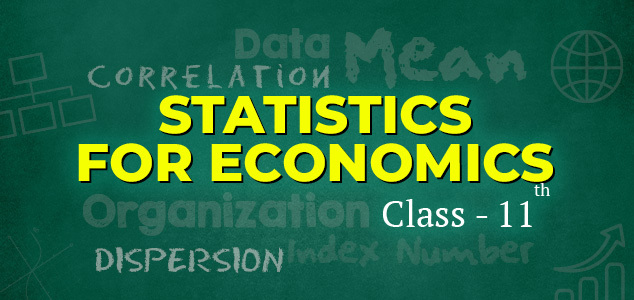Open in App
Not now

## Related Articles

• Write an Interview Experience
• CBSE Class 11 Statistics for Economics Notes

# CBSE Class 11 Statistics for Economics Notes

• Difficulty Level : Basic
• Last Updated : 03 Jan, 2023

Economic statistics is a topic in applied statistics that concerns the collection, organization, and presentation of data. GeeksforGeeks Class 11 Statistics for Economics Notes have been designed according to the CBSE Syllabus for Class 11. These revision notes consist of detailed Chapterwise important topics and concepts. Here, the learners can get easy access to the Chapterwise notes from the below-mentioned quick links. The notes contain 10 chapters covering every important topic, like Collection of Data, Organization of Data, Diagrammatic Presentation of Data, Mean, Median, Mode, Standard Deviation, Mean Deviation, and many more.### Chapter 1: Concept of Economics and Significance of Statistics in Economics

The first chapter of Class 11 Statistics for Economics is Concept of Economics and Significance of Statistics in Economics. The notes of this chapter contain the meaning of statistics in the plural and singular sense. Other important topics of this chapter are Functions, Importance and Limitations of Statistics.

### Chapter 2: Collection of Data

The second chapter of Class 11th Statistics for Economics is the Collection of Data. The notes of this chapter explain in detail how to collect data for further use and the different internal and external sources through which one can collect data. The important topics of this chapter contain Questionnaires, Qualities of a Good Questionnaire, Census Method of Collecting Data, Sampling Method, Statistical Errors, and many more. The notes explain the merits, demerits, and suitability of each of the Sources of Collecting Data.

### Chapter 3: Organisation of Data

Once the data is collected by the investigator, it needs to be properly organised for a better result. The third chapter of Class 11th Statistics for Economics is Organisation of Data, which covers important topics, like Classification of Data, Basis of Classification of Data, Statistical Series, Frequency Distribution, and Different Types of Frequency Distribution. Frequency Distribution is an important concept for a better understanding of further chapters.

### Chapter 4: Presentation of Data: Textual and Tabular

The collected and organised data is then presented in either textual, tabular, or diagrammatic form. The fourth chapter of Statistics for Economics covers the textual and tabular presentation of data. The notes explain the meaning, suitability, and drawbacks of textual presentation of data in detail, along with the tabular presentation and classification of data under tabular presentation.

• Textual Presentation of Data: Meaning, Suitability, and Drawbacks
• What do you know about Tabular Presentation of Data?
• What are the Different Kinds of Tables?
• Classification and Tabulation of Data

### Chapter 5: Diagrammatic Presentation of Data

The fifth chapter of Class 11th Statistics for Economics is Diagrammatic Presentation of Data. It explains in detail how an investigator can present the collected and organised data in the form of diagrams, like Pie Diagram, Bar Diagram, Histogram, Polygon, Ogive, and Arithmetic Line-Graph or Time-Series Graph. The notes consist of how to prepare these diagrams, along with an example for a better understanding of the topic.

### Chapter 6: Measures of Central Tendency: Arithmetic Mean

The sixth chapter of Class 11 Statistics for Economics is Measures of Central Tendency: Arithmetic Mean. The mean is the average of the given data set in either of the three series: individual, discrete and continuous. The notes of this chapter consist of Meaning, Types, Merits, and Demerits of Arithmetic Mean with an example for a better understanding. It also covers Simple Arithmetic Mean, Arithmetic Mean in Special Cases and Weighted Arithmetic Mean.

### Chapter 7: Measures of Central Tendency: Median and Mode

The next chapter of Class 11 Statistics for Economics also talks about Measures of Central Tendency. However, it covers Median and Mode. The notes of the seventh chapter consist of Meaning, Formula, Example, Merits, and Demerits of Median and Mode. Other important topics of this chapter are Graphical determination of Median, Partition Value, Graphic Method of Locating Mode, Relationship between Mean, Median and Mode.

### Chapter 8: Measures of Dispersion

The eighth chapter of Class 11 Statistics for Economics is Measures of Dispersion. The important topics under the notes of this chapter consist of Absolute and Relative Measures of Dispersion, Range, Quartile Deviation, Inter Quartile Range, Mean Deviation, Standard Deviation, Variance and Lorenz Curve. The notes explain each of the measures of dispersion in detail with their merits, demerits, formula, example and steps to calculate the measure.

### Chapter 9: Correlation

Correlation is the ninth chapter of Class 11 Statistics for Economics and covers important topics, like the meaning of correlation and three methods of calculating correlation. The three methods explained in the notes are the Scattered Diagram, Spearman’s Rank Correlation Coefficient and Karl Pearson’s Coefficient of Correlation (with steps, formula, and examples).

### Chapter 10: Index Number

The last chapter of Class 11th Statistics for Economics is Index Number. The notes of this chapter consist of important topics like Index Number, Methods of Constructing Index Number, Fisher’s Method, Laspeyre’s Method, and Paasche’s Method. It also explains Consumer Price Index, Wholesale Price Index, and Inflation in detail.

My Personal Notes arrow_drop_up